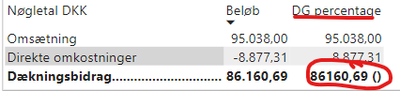cancel
Showing results for
Did you mean:

Fabric is Generally Available. Browse Fabric Presentations. Work towards your Fabric certification with the Cloud Skills Challenge.Helper II

## Calculate a percentage, based on separate rows

Hello community.

I need some help with creating a percentage based on buttom of a table and the top.I need it to take the 86.160,69 (Dækningsbidrag) and divide it by 95.038,00 (Omsætning) and shown as a percentage beside the number 86.160,69.

Any help would be appreciated.

1 ACCEPTED SOLUTIONSuper User

Hi @tobkres

``````Bel∅b Measure =
VAR NormalValue =
SUM ( TableName[Bel∅b] )
VAR OmstaetningValue =
CALCULATE (
SUM ( TableName[Bel∅b] ),
TableName[N∅glital DKK] = "OmstaetningValue"
)
RETURN
IF (
HASONEVALUE ( TableName[N∅glital DKK] ),
NormalValue,
NormalValue & " ("
& FORMAT ( DIVIDE ( NormalValue, OmstaetningValue ), "Percent" ) & ")"
)``````
5 REPLIES 5Helper II

Thank you for helping me out. I have tried, but i get the following error.

``Column 'Inversbeloeb' in table 'MeasureTable' cannot be found or may not be used in this expression.``

This is the code:

``````DG percentage =
VAR NormalValue =
SUM ( MeasureTable[Inversbeloeb] )
VAR RevenueValue =
CALCULATE(
SUM ( MeasureTable[Inversbeloeb] ),
Sortorder[KontoOverskrift] = "RevenueValue"
)
RETURN
IF (
HASONEVALUE ( Sortorder[KontoOverskrift] ),
NormalValue,
NormalValue & " ("
& FORMAT ( DIVIDE ( NormalValue, RevenueValue ), "Percent" ) & ")" ``````

These are the fields.Have i missed something?Super User

@tobkres

``````DG percentage =
VAR NormalValue = [Inversbeloeb]
VAR RevenueValue =
CALCULATE ( [Inversbeloeb], Sortorder[KontoOverskrift] = "RevenueValue" )
RETURN
IF (
HASONEVALUE ( Sortorder[KontoOverskrift] ),
NormalValue,
NormalValue & " ("
& FORMAT ( DIVIDE ( NormalValue, RevenueValue ), "Percent" ) & ")"
)``````Helper II

Seems like it just returns the same numbers.Super User

@tobkres
Please make sure you are using the correct spilling. Copy the name from inside the column. If you want we can connect via zoom. Will take only 10min to sort it out.Super User

Hi @tobkres

``````Bel∅b Measure =
VAR NormalValue =
SUM ( TableName[Bel∅b] )
VAR OmstaetningValue =
CALCULATE (
SUM ( TableName[Bel∅b] ),
TableName[N∅glital DKK] = "OmstaetningValue"
)
RETURN
IF (
HASONEVALUE ( TableName[N∅glital DKK] ),
NormalValue,
NormalValue & " ("
& FORMAT ( DIVIDE ( NormalValue, OmstaetningValue ), "Percent" ) & ")"
)``````Announcements#### Power BI Monthly Update - November 2023

Check out the November 2023 Power BI update to learn about new features.#### Fabric Community News unified experience

Read the latest Fabric Community announcements, including updates on Power BI, Synapse, Data Factory and Data Activator.#### The largest Power BI and Fabric virtual conference

130+ sessions, 130+ speakers, Product managers, MVPs, and experts. All about Power BI and Fabric. Attend online or watch the recordings.Top Solution Authors
Top Kudoed Authors
Users online (2,419)